Students can download 11th Economics Chapter 2 Consumption Analysis Questions and Answers, Notes, Samcheer Kalvi 11th Economics Guide Pdf helps you to revise the complete Tamilnadu State Board New Syllabus, helps students complete homework assignments and to score high marks in board exams.

## Tamilnadu Samacheer Kalvi 11th Economics Solutions Chapter 2 Consumption Analysis

### Samacheer Kalvi 11th Economics Consumption Analysis Text Book Back Questions and Answers

Part – A

Multiple Choice Questions:

Question 1.
Pick the odd one out …………………….
(a) Luxuries
(b) Comforts
(c) Necessaries
(d) Agricultural goods
(d) Agricultural goodsQuestion 2.
The choice is always constrained or limited by the ________ of our resources.
(a) Scarcity
(b) Supply
(c) Demand
(d) Abundance
(a) Scarcity

Question 3.
The chief exponent of the Cardinal utility approach was ……………………..
(a) JR Hicks
(b) R G D Allen
(c) Marshall
(d) Stigler
(c) Marshall

Question 4.
Marginal Utility is measured by using the formula of
(a) TUn -TUn-1
(b) TUn-TUn+1
(c) TUn+TUn+1
(d) TUn-TUn+1
(a) TUn -TUn-1Question 5.
When marginal utility reaches zero, the total utility will be …………………..
(a) Minimum
(b) Maximum
(c) Zero
(d) Negative
(b) Maximum

Question 6.
Gossen’s first law is known as.
(a) Law of Equi-marginal utility.
(b) Law of diminishing marginal utility
(c) Law of demand.
(d) Law of Diminishing returns.
(b) Law of diminishing marginal utility

Question 7.
The basis for the law of demand is related to ……………………..
(a) Law of diminishing marginal utility
(b) Law of supply
(c) Law of equi-marginal utility
(d) Gossen’s Law
(a) Law of diminishing marginal utilityQuestion 8.
The concept of consumer’s surplus is associated with
(b) Marshall
(c) Robbins
(d) Ricardo
(b) Marshall

Question 9.
Given potential price is Rs.250 and the actual price is Rs.200. Find the consumer surplus ……………………….
(a) 375
(b) 175
(c) 200
(d) 50
(d) 50Question 10.
Indifference curve approach is based on
(a) Ordinal approach
(b) Cardinal approach
(c) Subjective approach
(d) Psychological approach
(a) Ordinal approach

Question 11.
The concept of elasticity of demand was introduced by …………………….
(a) Ferguson
(b) Keynes
(d) Marshall
(d) Marshall

Question 12.
Increase in demand is caused by
(a) Increase in tax
(b) Higher subsidy
(c) Increase in interest rate
(d) decline in population
(b) Higher subsidyQuestion 13.
The movement on or along the given demand curve is known as ………………………..
(a) Extension and contraction of demand
(b) Shifts in the demand
(c) Increase and decrease in demand
(d) All the above
(a) Extension and contraction of demand

Question 14.
In case of relatively more elastic demand, the shape of the curve is
(a) Horizontal
(b) Vertical
(c) Steeper
(d) Flatter
(d) Flatter

Question 15.
A consumer is in equilibrium when marginal utilities from two goods are …………………….
(a) Minimum
(b) Inverse
(c) Equal
(d) Increasing
(c) EqualQuestion 16.
Indifference curve was first introduced by
(a) Hicks
(b) Allen
(c) Keynes
(d) Edgeworth
(d) Edgeworth

Question 17.
Elasticity of demand is equal to one indicates ………………………
(a) Unitary Elastic Demand
(b) Perfectly Elastic Demand
(c) Perfectly Inelastic Demand
(d) Relatively Elastic Demand
(a) Unitary Elastic Demand

Question 18.
The locus of the points which gives the same level of satisfaction is associated with
(a) Indifference Curves
(b) Cardinal Analysis
(c) Law of Demand
(d) Law of Supply
(a) Indifference CurvesQuestion 19.
Ordinal utility can be measured by …………………………
(a) Ranking
(b) Numbering
(c) Wording
(d) None of these
(a) Ranking

Question 20.
The indifference curve is
(a) Vertical
(b) Horizontal
(c) Positive sloped
(d) Negatively sloped
(d) Negatively sloped

PART – B

Answer the following in one or two sentences.

Question 21.
Define Utility?
The utility is the capacity of a commodity to satisfy human wants.Question 22.
Mention the classification of wants?
Wants are broadly classified into three categories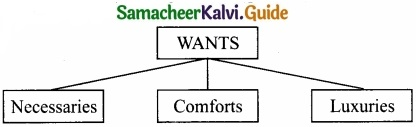1. Necessaries: Goods which are indispensable for human beings to exist in the world are called “Necessaries”. For example, food, clothing, and shelter.
2. Comforts: Goods which are not indispensable for life but to make our life easy, convenient and comfortable are called “Comforts”. Ex: TV, Fan, Refrigerator and Air conditioner.
3. Luxuries: Goods which are not very essential but are very costly are known as “Luxuries”. Ex: Jewellery, Diamonds, and ars. However, for people with higher income, they may look necessaries or comforts.

Question 23.
Name the basic approaches to consumer behavior.
There are two basic approaches, namely:
1. Utility approach

• The utility approach involves the use of measurable (cardinal) utility to study consumer behaviour.
• Marshall is the chief exponent of the utility approach to the theory of demand. It is known as cardinal utility analysis or Marginal utility analysis or Marshallian utility analysis.

2. Indifference curve approach

• The indifference curve approach was the idea of comparable utility [ordinal utility] J.R. Hicks and R.G.D. Allen introduced the indifference curve approach.Question 24.
What are the degrees of price elasticity of demand?
1. The price elasticity of demand, commonly known as the elasticity of demand refers to the responsiveness and sensitiveness of demand for a product to the changes in its price. In other words, the price elasticity of demand is equal to
EP =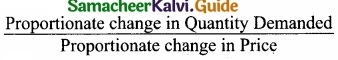Numerically, EP = $$\frac { \Delta Q }{ \Delta P }$$ × $$\frac{P}{Q}$$
where, ∆Q = Q1 – Q0, ∆P = P1 – P0
Q1 = New quantity, P1 = New price
Q0 = Original quantity, P0 = Original price

Question 25.
State the meaning of indifference curves.

1. The Consumer is rational and his aim is to derive maximum satisfaction.
2. The utility cannot be cardinally measured but can be ranked or compared or ordered by an ordinal number such as I, II, III, and so on.
3. The indifference curve approach is based on the concept “Diminishing Marginal Rate of Substitution”.
4. The consumer is consistent. This assumption is called the assumption of transitivity.Question 26.
Write the formula of consumer surplus?
Consumer’s Surplus = Total utility
[Actual price × Units of commodity]
– TU – [P × Q]
= 20 – [2 × 5] = 20 – 10 = 10
Total utility = 20
Actual price = 2
Quantity = 5

Question 27.
What are Giffen goods? Why it is called that?
Giffen Paradox: The Giffen good or inferior good is an exception to the law of demand. When the price of inferior goods, falls, the poor will buy less and vice versa.

Part – C

Answer the following questions in one paragraph.

Question 28.
Describe the feature of human wants?
In ordinary language desire and want mean the same thing. But in economics, they have different meanings. Wants are the basis for human behaviour to buy and consume goods.

Characteristics of Human Wants:

• Wants are unlimited: Human wants are countless in number and various in kinds. When one wants is satisfied another wants to crop up. Human wants to multiply with the growth of civilization and development.
• Wants become habits: Wants become habits; for example, when a man starts reading the newspaper in the morning, it becomes a habit. The same is the case with drinking tea or chewing pans.
• Wants are Satiable: Though we cannot satisfy all our wants, at the same time we can satisfy particular wants at a given time. When one feels hungry, he takes food and that want is satisfied.
• Wants are Alternative: There are alternative ways to satisfy a particular want eg. Idly, dosa or chappati.
• Wants are Competitive: All our wants are not equally important. So, there is competition among wants. Hence, we have to choose more urgent wants than less urgent wants.
• Wants are Complementary: Sometimes, the satisfaction of a particular want requires the use of more than one commodity. Example: Car and Petrol, Ink and Pen.
• Wants are Recurring: Some wants occur again and again. For example, if we feel hungry, we take food and satisfy our wants. But after some time, we again feel hungry and want food.Question 29.
Mention the relationship between marginal utility and total utility?

 Marginal utility Total utility 1. Marginal utility goes on diminishing. 1. Total utility goes on Increasing. 2. Marginal utility becomes zero. 2. Total utility maximum. 3. Marginal utility becomes negative. 3. Total utility diminishes.

Question 30.
Explain the concept of consumer’s equilibrium with a diagram?
Consumer Equilibrium: The consumer reaches equilibrium at the point where the budget line is tangent on the indifference curve.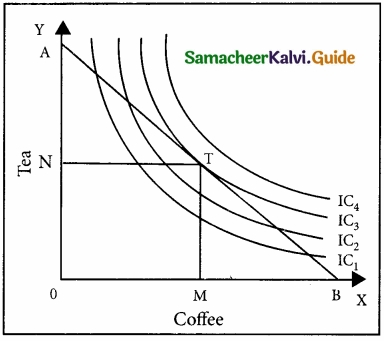‘T’ is the point of equilibrium as budget line AB is tangent on indifference curve IC3 the uppermost IC which implies the maximum possible level of satisfaction.
At equilibrium point, the slope of IC refers to MRSxy and the slope of BL (Budget Line) refers to the ratio of the price of x to the price of y.
i.e., $$\frac { P_{ x } }{ P_{ y } }$$, Therefore MRSxy = $$\frac { P_{ x } }{ P_{ y } }$$Question 31.
Explain the theory of “consumer’s surplus”
Alfred Marshall defines consumer’s surplus as “the excess of price which a person would be willing to pay a thing rather than go without the thing, over that which he actually does pay is the economic measure of this surplus satisfaction. This may be called consumer’s surplus”.

Question 32.
Distinguish between extension and contraction of demand?

1. The changes in the quantity demanded a commodity due to the change in its price alone are called “ Extension and Contraction of demand”.
2. Buying more at a lower price and less at a higher price is known as “ Extension and Contraction of Demand”.Question 33.
What are the properties of indifference curves?
Indifference curves are subjective and unique to each person. Nevertheless, they have in common the following properties:

1. Indifference curve must have negative slope:
An indifference curve has a negative slope, which denotes that if the quantity of a commodity (y) decreases, the quantity of the other (x) must increase if the consumer is to stay on the same level of satisfaction.

2. Indifference curves are convex to the origin:
Indifference curves are not only negatively sloped but are also convex to the origin. The convexity of the indifference curves implies that not only two commodities are substitutes for each other but also the fact that the marginal rate of substitution (MRS) between the goods decreases as a consumer moves along an indifference curve.

3. Indifference curve cannot intersect:
IC1 is a lower indifference curve denoting lesser satisfaction. Combination C and B Fallon IC1.
IC2 is the upper indifference curve denoting higher satisfaction. C and A combinations are on IC2

At the point of intersection, C = B on IC1 and C = A on IC2. So A = B whereas, A is in upper IC and B is on lower IC.
This is not possible.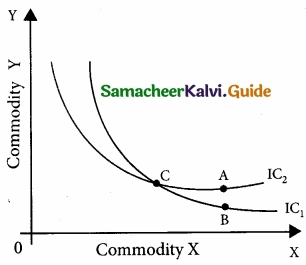4. Indifference curves do not touch the horizontal or vertical axis.
If they touch the axis, it violates the basic assumption that the consumer purchases two commodities in a combination.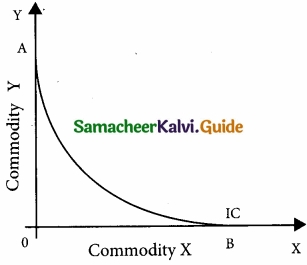Question 34.
Briefly explain the concept of consumer’s equilibrium?
Consumer’s equilibrium refers to a situation under which a consumer spends his entire income on the purchase of goods, in such a manner that it gives him maximum satisfaction.
The consumer reaches equilibrium at the point where the budget line is tangent on the indifference curve.
T is the point of Equilibrium as budget line AB is tangent on indifference curve IC3, the upper IC implies a maximum level of satisfaction.

Part – D

Question 35.
Explain the law of demand and its exceptions?
Definitions:
The Law of Demand says as “the quantity demanded increases with a fall in price and diminishes with a rise in price”. – Marshall
“The Law of Demand states that people will buy more at lower price and buy less at higher prices, other things remaining the same”. – Samuelson Assumptions of Law of Demand:

1. The income of the consumer remains constant.
2. The taste, habit and preference of the consumer remain the same.
3. The prices of other related goods should not change.
4. There should be no substitutes for the commodity in the study.
5. The demand for the commodity must be continuous.
6. There should not be any change in the quality of the commodity’.

Given these assumptions, the law of demand operates. If there is change even in one of these assumptions, the law will not operate.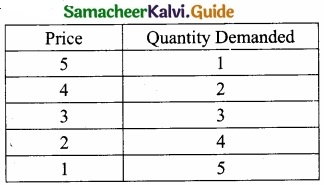Explanation:
The law of demand explains the relationship between the price of a commodity and the quantity demanded of it. This law states that the quantity demanded of a commodity expands with a fall in price and contracts with a rise in price. In other words, a rise in the price of a commodity is followed by a contraction demand, and a fall in price is followed by an extension in demand. Therefore, the law of demand states that there is an inverse relationship between the price and the quantity demanded of a commodity.

Exceptions to the law of demand:
Normally, the demand curve slopes downwards from left to right. But there are some unusual demand curves which do not obey the law and the reverse occurs. A fall in price brings about a contraction of demand and a rise in price results in an extension of demand. Therefore the demand curve slopes upwards from left to right. It is known as the exceptional demand curve.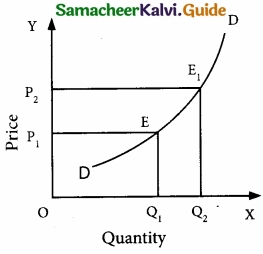In the above diagram, DD is the demand curve which slopes upwards from left to right. It shows that when the price is OP1 OQ1, is the demand and when the price rises to OP2, demand also extends to OQ2.Question 36.
Elucidate the law of diminishing marginal utility with a diagram?
Introduction:
H.H.Gossen, an Austrian Economist was the first to formulate this law in Economics in 1854. Therefore Jevons called this law “ Gossen’s First Law of Consumption”. But credit goes to
Marshall, because he perfected this law on the basis of Cardinal Analysis. This law is based on the characteristics of human wants, i.e. wants are satiable.

Definition:
Marshall states the law as “ the additional benefit which a person derives from a given increase of his stock of a thing, diminishes with every increase in the stock that he already has”.

Assumptions:

1. The utility can be measured by cardinal numbers such as 1, 2, 3, and so on.
2. The marginal utility of money of the consumer remains constant.
3. The consumer should be a rational consumer and his aim is to attain maximum satisfaction .with minimum expenditure.
4. The units of the commodity consumed must be reasonable in size.
5. The commodity consumed should be homogeneous or uniform in character like weight, quality, taste, colour etc.
6. The consumption of goods must take place continuously at a given period of time.
7. There should be no change in the taste, habits preferences, fashions, income, and character of the consumer during the process of consumption.

Explanation:
The Law of Diminishing Marginal utility states that if a consumer continues to consume more and more units of the same commodity, its marginal utility diminishes. This means that the more we have of a thing, the less is the satisfaction or utility that we derive from the additional unit of it.

The Law of Diminishing Marginal utility: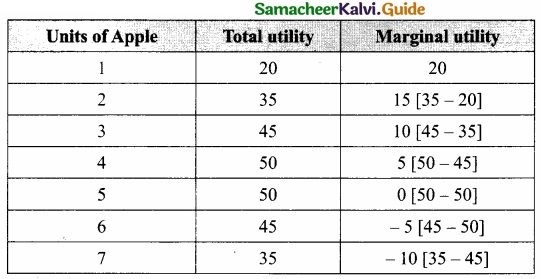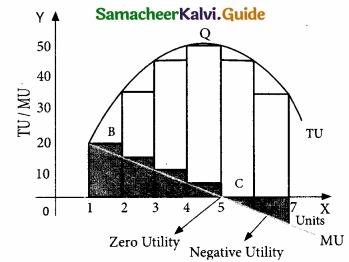1. In this table, we find that the total utility goes on increasing but at a diminishing rate.
2. The law can be explained with a simple illustration.
3. The consumer wants to consume 7 apples one after another.
4. The utility from the first apple is 20.
5. But the utility from the second apple will be less than that of the first [say 15].
6. The third less than that of the second [say 10] and so on.
7. Finally, the utility from the fifth apple becomes zero, and the utilities from the sixth and seventh apples are negative for disutility or disliking.
8. This tendency is called the “ The Law of Diminishing Marginal Utility”.
9. In this table we find on the other hand, marginal utility goes on diminishing.
10. When marginal utility becomes zero, the total utility is maximum and when marginal utility becomes negative, the total utility diminishes.

The X-axis represents the number of apples consumed
Y-axis represents total utility and marginal utility
TU – represents total utility
MU – represents marginal utility

Criticisms:

1. The utility cannot be measured numerically, because the utility is subjective.
2. This law is based on unrealistic assumptions.
3. This law is not applicable to indivisible commodities.Question 37.
Explain the law of Equi – marginal utility?
The law of the Equi-marginal utility:

1. The law of diminishing marginal utility is applicable only to the want of a single commodity.
2. The law of equity – marginal utility explains the behavior of a consumer when he consumes more than one commodity.
3. Wants are unlimited but the income which is available is limited.
4. This law explains how the consumer spends his limited income on various commodities to get maximum satisfaction.
5. Law of Equi-Marginal Utility is also known as “The Law of Substitution” (or) “The Law of Consumer’s Equilibrium ” (or) “Gossen’s Second Law” and “ The Law of Maximum Satisfaction”.

Definition:
Marshall states the law as,“ If a person has a thing which he can put to several uses, he will distribute it among these uses in such a way that it has the same marginal utility in all. For, if it had a greater marginal utility in one use than another he would gain by taking away some of it from the second use and applying it to first”.

Assumptions:

1. The consumer is rational in the sense that he wants to get maximum satisfaction.
2. The utility of each commodity is measurable in cardinal numbers.
3. The marginal utility of money remains constant.
4. The income of the consumer is given.
5. There is perfect competition in the market.
6. The prices of the commodities are given.
7. The law of diminishing marginal utility operates.

Illustration:
This Law can be illustrated with the help of a table. Let us assume that the consumer has a given income of ₹11. He wants to spend this entire income [i.e. ₹11 ] on Apple and Orange. The price of an Apple and the price of an Orange is each.

The law of the Equi – Marginal Utility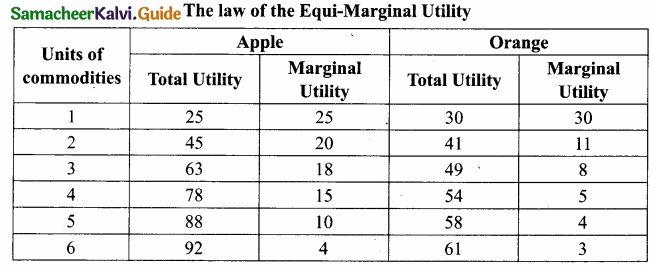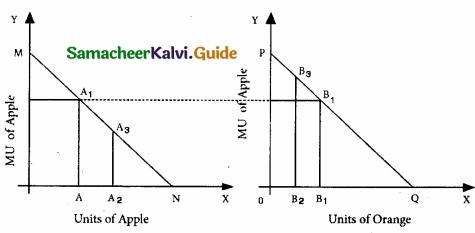If the consumer wants to attain maximum utility, he should buy 6 units of apples and 5 units of oranges, so that she can get [92 + 58] = 150 units. No other combination of Apple and Orange can give higher than 150 utilities. $$\frac{MUA}{PA}$$ = $$\frac{MUD}{PO}$$ i.e; $$\frac{4}{1}$$ = $$\frac{4}{1}$$

Here,

Diagrammatic Illustration:

1. In the diagram, X-axis represents the amount of money spent and Y-axis represents the marginal utilities of Apple and Orange respectively.
2. If the consumer spends ₹6 on Apple and ₹5 on Orange, the marginal utilities of both are equal, i.e. AA1 = BB1 [4 = 4], Hence, he gets maximum utility.

Criticisms:

1.  In practice, the utility cannot be measured, only be felt.
2. This law cannot be applied to durable goods.Question 38.
What are the methods of measuring the Elasticity of demand?
There are three methods of measuring the price elasticity of demand.

1. The Percentage method: ep = $$\frac{∆Q}{∆P}$$ × $$\frac{P}{Q}$$
It is also known as ratio method, when we measure the ratio as:
ep = $$\frac { %\Delta Q }{ %\Delta P }$$ where,
% ∆Q = Percentage change in demand
% ∆P = Percentage change in price

2. Total Outlay Method:
Marshall suggested that the simplest way to decide whether demand is elastic or inelastic is to examine the change in total outlay of the consumer or total revenue of the firm. Total Revenue = (Price × Quantity Sold)
TR = ( P × Q)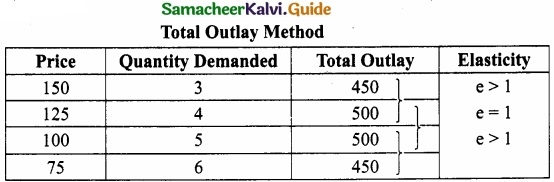Where there is an inverse relation between Price and Total Outlay, demand is elastic. Direct relation means inelastic. Elasticity is unity when Total Outlay is constant.

3. Point or Geometrical Elasticity:
When the demand curve is a straight line, it is said to be linear. Graphically, the point elasticity of a linear demand curve is shown by the ratio of the segments of the line to the right and to the left of the particular point. Where ‘e’ stands for point elasticity, ‘L’ stands for the lower segment, and ‘U’ for the upper segment.

Point Elasticity =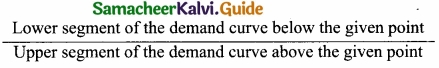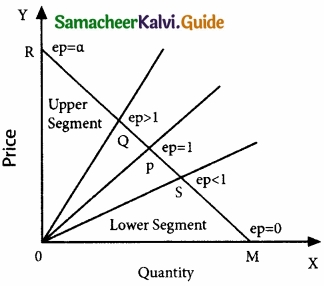ep = L/U

Where ‘ep’ stands for point elasticity, ‘L’ stands for the lower segment, and ‘U’ for the upper segment.

### Samacheer Kalvi 11th Economics Consumption Analysis Additional Important Questions and Answers

PART – A

Multiple Choice Questions:

Question 1.
_________ is the beginning of Economic Science.
(a) Production
(b) Consumption
(c) Exchange
(d) Distribution
(b) ConsumptionQuestion 2.
Single commodity consumption mode is ………………………
(a) Production possibility curve
(b) Law of Equi – marginal utility
(c) Law of supply
(d) Law of diminishing marginal utility
(d) Law of diminishing marginal utility

Question 3.
If total utility is maximum, then marginal utility is _________.
(a) Negative
(b) Zero
(c) Positive
(d) Maximum
(b) ZeroQuestion 4.
An indifference curve is ……………………. to the origin.
(a) Convex
(b) Concave
(c) Narrow
(d) Cardinal
(a) Convex

Question 5.
‘Diamond – Water paradox’ was given by
(a) Marshall
(b) Robbins
(d) Samuelson

Question 6.
Human wants to have the capacity to get satisfied only ……………………..
(a) Permanent
(b) Substitute
(c) Satisfaction
(d) Temporarily
(d) TemporarilyQuestion 7.
The exceptional demand curve _________.
(a) Slopes downward
(b) Vertical
(c) Slopes upward
(d) Horizontal
(c) Slopes upward

Question 8.
What is called conventional necessities?
(a) Comforts
(b) Luxuries
(c) Necessaries
(d) Necessary
(a) ComfortsQuestion 9.
Mathematically consumer’s surplus is _________
(a) TU-TU
(b) TR- (P x Q)
(c) TU – (P x Q)
(d) TC – (Q x P)
(c) TU – (P x Q)

Question 10.
What is the other name for the law of equity-mangìnal utility?
(a) Gossen’s First law
(b) Gossen’s Second law
(c) Gossen’s Third law
(d) Gossen’s Fourth law
(b) Gossen’s Second law

Part – B

Answer the following in one-two sentences.

Question 1.
What are the criticisms of the law of equity-marginal utility?

1. The utility cannot be measured, only be felt.
2. This law cannot be applied to durable goods.Question 2.
What is Human wants conditions?
Human wants are countless in number and various in kinds. When one want is satisfied another want crops up. Human wants to multiply with the growth of civilization and development.

Question 3.
Mention the types of elasticity of demand.

1. Price elasticity of demand.
2. Income elasticity of demand
3. Cross elasticity of demand.

Part – C

Answer the following questions in one paragraph.

Question 1.
Write a note on the Price line or Budget line?
Demand for a good depends upon (z) preference for that good and (z’z) purchasing power.
The preference pattern is represented by a set of indifference curves. The purchasing power depends on his money income and the price of the goods. The money income and price level are represented by the budget line. The budget line is a downward sloping straight line connecting X-axis and Y-axis as follows.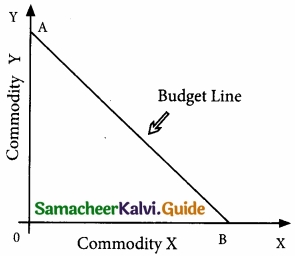OA- income, OA/OB price of X good. The budget line is the line joining various combinations of the two goods which the consumer can buy at given prices and income.Question 2.
Enumerate the characteristics of demand.
Price: Demand is always related to price.
Time: Demand always means demand per unit of time, per day, per week, per month or per year
Market: Demand is always related to the market, buyers, and sellers.
Amount: Demand is always a specific quantity that a consumer is willing to purchase.

Part – D

Question 1.
Explain the Determinants of Elasticity of demand?
There are many factors that determine the degree of price elasticity Of demand. Some of them are described below:

1. Availability of Substitutes:
If close substitutes are available for a product, then the demand for that product tends to be very elastic. If the price of that product increases, buyers will buy its substitutes; hence fall in its demand will be very large. Hence, price elasticity will be larger, e.g., Vegetables.

For salt no close substitutes are available. Hence even if the price of salt increases the fall in demand may be zero or less. Hence salt is price inelastic.

2. Proportion of consumer’s income is spent’ if the smaller proportion of consumer’s income is spent on particular commodity say X, price elasticity of demand for X will be smaller. Take for example salt, people spend a very small proportion of their income on salt. Hence, the salt will have small elasticity of demand, or inelastic.

3. Number of uses of commodity:
If a commodity is used for a greater number of uses, its price elasticity will also be larger. For example, milk is used as buttermilk, curd, ghee, and for making ice cream, etc. Hence, even the small fall in the price of milk will tempt the consumers to use more milk for many purposes. Hence milk has a greater price elasticity of demand.

4. Complementarity between goods:
For example, along with petrol, lubricating oil is also used for running automobiles. Here a rise in the price of lubricating oil may not reduce the demand for lubricating oil. Hence, the complementary good, here, lubricating oil, will be price inelastic.

5. Time:
In the long run, the price elasticity of demand for many goods will be larger. This is so because, in the long run many substitutes can be discovered or invented. Therefore, the demand is generally more elastic in the long run, than in the short run. In the short run bringing out new substitutes is difficult.Question 2.
What is the Importance of Elasticity of demand?